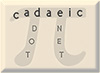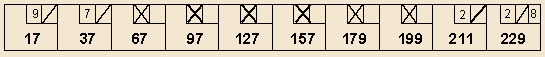Bowling for Primes Mike Keith March 1999

Here is an interesting puzzle combining a recreation not normally thought of as very mathematical (bowling) and something quite mathematical (viz., the prime numbers). [This puzzle - not the solution - came to me in toto during a dream, a fact which I leave to the experts on the subconscious to analyze.]

Puzzle: what's the highest you can score in a standard game of bowling if your score in each of the 10 frames is required to be a prime number?

So as not to spoil the puzzle by immediately giving the answer (which we do give later on this page), we first discuss the generalization to n frames. In this version, we redefine the game of bowling to consist of n frames rather than 10, with the last frame containing extra balls if necessary, as usual, if they are required to determine the scoring of the last frame. What's the answer to our question for each value of n?

Well, n=1 is very easy: two strikes followed by a 9, in the one and only frame of the game, gives a prime score of 29, which is clearly the largest possible since it's the largest prime < 30 (the maximum score per frame in bowling).

The case n=2 is not so trivial. Suppose we start, as in n=1, by scoring 29 in the first frame. The second (incomplete) frame then has X 9 (a strike followed by a 9), which can only be completed in two ways: by picking up the last pin to get a spare (which yields a score of 49) or by missing the last pin (which yields a score of 48). Since neither of these is prime, we see that the greedy algorithm does not work. Recall that a greedy algorithm is one that attempts to score the most in the first frame, then given that to score the most in the second, and so on. The failure of the greedy algorithm for n=2 is an indication that it will probably fail for most larger values of n as well.

The next best score we can get in the first frame is 23 (since 25 and 27 are not primes). We can follow this (X X 3) by knocking down the remaining 7 pins for a spare, thus giving 43 (also prime) in the second frame. Thus the answer for n=2 is 43.

The best scores achievable for n=1 to 9 are:

 n Best score 1 29 2 43 3 67 4 97 5 127 6 157 7 181 8 199 9 211

The function score(n)/n is interesting - it starts at its maximum of 29, then dips but recovers to a local maximum of 26 and 1/6 at n=6, then starts to decrease again. What happens for larger n? Does it ever recover and get above 26+1/6 again? (We somewhat tentatively conjecture "no".) Can you extend this table to larger n?

Here is one way (there are others) to score 229, the maximum possible, in the standard 10-frame game:(Another interesting way to do it is to change the first two frames to 7- X, which causes the game to have 7 strikes in a row - the maximum possible in an all-prime game.) The proof that this is the maximum possible score seems to be not simple; our best proof uses computer assistance and so is not easily proffered in words. The success of the above game is dependent on a nice long string of small primes in arithmetic progression with difference 30 (7, 37, 67, 97, 127, 157).

Real bowlers will note that in the last two frames of this game the first ball scores two pins. It is, in fact, nearly impossible to knock down just two pins on the first ball (one and three are quite easy, but not two), so we can make a whole new variation on this problem in which a score of 2 on the first ball is disallowed. We leave it as an exercise to the reader to show that 223 is the maximum possible in a 10-frame game for this somewhat more realistic version of the problem.

Another related question is: suppose a game can last for an unlimited number of frames (but still, with the rule that all frames must have a prime score). Now what is the highest possible score? It's immediately obvious that more that 1327 is not possible, because the next prime after 1327 is 1361, which has a gap of more than 30. It's also fairly easy to construct a (possibly very long) game that scores 1327. So the real question is: what's the shortest possible game that scores 1327?

Dave Boll reports the following game found by his colleague Dave Boyd. It scores 1327 in 59 frames:

```X   |  X   | 3 /  | 8 /  | 8 /  | 8 /  | 6 /  | 8 /  |  X   |  X   |
23     43     61     79     97    113    131    151    181    211

X   | X    |  X   |  X   | 2 /  | 8 /  | 8 /  | 0 /  |  X   |  X   |
241    271    293    313    331    349    359    379    409    439

X   |  X   | 8 /  | 6 /  | 8 /  |  X   |  X   |  X   |  X   |  X   |
467    487    503    521    541    571    601    631    661    691

X   |  X   | 8 2/ | 2 4  |  X   |  X   |  X   | 2 /  | 0 /  | 8 /  |
719    739    751    757    787    809    829    839    857    877

X   |  X   |  X   |  X   |  X   |  X   | 2 0  |  X   |  X   |  X   |
907    937    967    997   1019   1031   1033   1063   1093   1123

X   |  X   |  X   | 8 /  | 6 /  | 2 /  |  X   |  X   |X 8 /
1153  1181   1201   1217   1229   1249   1279   1307   1327```

This construction was the result of a few hundred hours of computer searching using a smart algorithm. Dave also attempted to find a 58-frame game without success, so it is believed (fairly strongly) that 59 is the minimum possible. The same question with the first-roll-of-2-disallowed rule is still open (since the game above has several such frames).